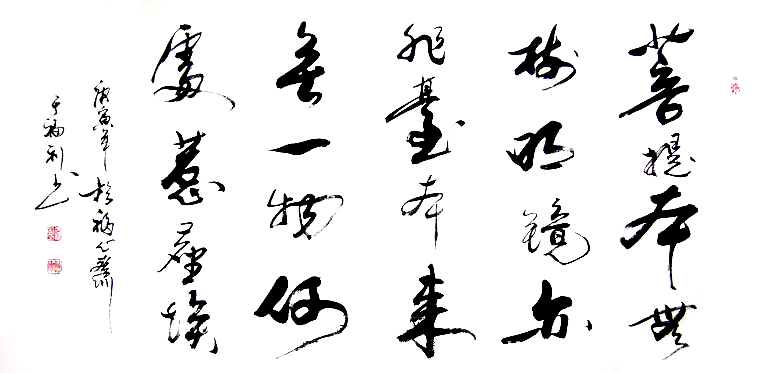# 聆听纯净心音

• 绿度母心咒（伊藤佳代）
• 佛陀牵着我的手（李佳宁）
• 三德歌（谭晶）
• 放生歌（郭乔伊）
• 大悲咒（邝美云）
• 普贤行愿品偈颂（朱震颐）
• 菩提梵唱（郭乔伊）
• 禅境（桑吉平措）
• 做个好孩子 学唱弟子规（敬善媛）
• Mere Raam（Snatam Kaur）
• 文殊菩萨心咒（敬善媛）
• 半壶纱（刘珂矣）
• 万物生（梵文）
• 相见（桑吉平措）
• 天之大（韩红）
• 赞佛偈（姚斯婷）
• 六字大明咒（敬善媛）
• 地藏菩萨灭定业真言（姚斯婷）
• 悟（邓伟标）
• 准提神咒（敬善媛）
• 佛陀的爱（许俊华）
• 药师心咒（般禅梵唱妙音组）
• 春（五台山普寿寺梵乐小组）
• 人间净土（方颖）
• 生命（韩红）
• 水流众生（李键）
• 心经（王菲）
• 南无阿弥陀佛（李娜）
• 玛智（尕让邓真）
• 象雄传说（尕让邓真）
• 河流（汪峰）
• 禅是一枝花（龚玥）
• 点灯祈愿文（张杰）
• 梦中的额吉（乌达木）
• 为你祈祷（魏新雨）
• 莲师心咒 (舒缓版)（马常胜）
• 白度母心咒（马常胜）
• 菩提（李玉刚）
• 喇嘛仟（阿斯根）
• 琵琶语（纯音乐）
• 花为谁开（祺云法师）
• 四季（纳兰）
• 知否知否（胡夏、郁可唯）
• 禅 - 纯音乐（戴亚）
• 我的楼兰（云朵）
• 静虑 - 纯音乐（戴亚）
• 有一份爱（黑鸭子合唱组）
• 四无量心（姚斯婷）
• 缘起（姚斯婷）
• 极乐世界是我家（任静&付笛声）
• 发菩提心真言（姚斯婷）
• 金刚萨埵百字明（甲雍）
• 释迦牟尼佛心咒（甲雍）
• 黄财神心咒（甲雍）
• 文殊心咒（甲雍）
• 五路财神祈愿招财经（甲雍）
• 药师佛心咒（甲雍）
• 大白伞盖佛母心咒（甲雍）
• 观音菩萨悲赞（桑吉平措）
• 阿弥陀佛在心间（桑吉平措）
• 文殊菩萨赞（桑吉平措）
• 忏悔文（桑吉平措）
• 增福歌（桑吉平措）
• 莲心曲（敬善媛）
• 大悲咒 - 少林问道（敬善媛）
• 心经 - 新西游记（敬善媛）
• 南无观世音菩萨（敬善媛）
• 稽山梵音（王珂迩）
• 静水流深 - 纯音乐（巫娜）